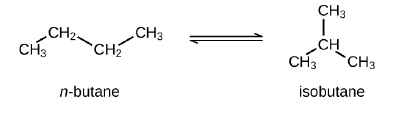# Problem: Butane exists as two isomers, n−butane and isobutane.KP = 2.5 at 25 °CWhat is the pressure of isobutane in a container of the two isomers at equilibrium with a total pressure of 1.22 atm?

###### FREE Expert Solution

Recall: The equilibrium constant is the ratio of the pressure or concentration of products and reactants, with each compound being raised to a power equivalent to the stoichiometric coefficient in the chemical equation.

For the reaction aA + bB ⇌ cC + dD, the equilibrium constant is:where Kp is used for pressures and Kc is used for concentrations.

The Kp expression for the isomerization of n-butane is:94% (135 ratings)###### Problem Details

Butane exists as two isomers, n−butane and isobutane.

KP = 2.5 at 25 °C
What is the pressure of isobutane in a container of the two isomers at equilibrium with a total pressure of 1.22 atm?